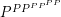# Complexity of square-root sum

 Importance: Medium ✭✭
 Author(s): Goemans, Michel (?)
 Subject: Theoretical Computer Science » Complexity
 Keywords: semi-definite programming
 Posted by: abie on: July 18th, 2007
Question   What is the complexity of the following problem?

Given, determine whether or notAs of a 1998 survey, the complexity of this problem was unknown. I'm not sure if that's still the case. But I wanted to see how easy it was to make a page about it.

This is the key to determining if semi-definite programming is truly solvable in polynomial time (it can be approximated to withinusing the interior point method or the ellipsoid algorithm in time polynomial in the size of the instance and.

## Bibliography

[G] Michal Goemans, Semidefinite Programming and Combinatorial Optimization

* indicates original appearance(s) of problem.

### Complexity

The complexity of the problem as stated is worst case O(n^3) in the magnitude of the greatest value a[i]. The reason being that it is a simple sum, as stated, of square roots. The square root operation is O(n^2) in the number of bits, which has a 3.xxx:1 relation to the magnitude of each a[i]. Either the problem is mistated or it has not been significant enough for anyone to waste time on stating the obvious.

### Filching 10:00(2)

I think the problem is that you may need to compute square roots to high precision. If you need to know the first m digits in the decimal expansion of sqrt(5), it will probably take time linear in m to find them, even though 5 took only constantly many bits to encode.

### A slightly more general

A slightly more general problem (in which the square roots may be subtracted as well as added) is very important in the context of computational geometry, both for actual algorithms and for proving complexity-theoretic bounds on problems, as it encapsulates the difficulty of comparing lengths of polygonal chains; see http://maven.smith.edu/~orourke/TOPP/P33.html.
—David Eppstein

### Some update

Quotation from Etessami and Yannakakis, 2007:

\item [Sqrt-sum problem] is known to be solvable in PSPACE but it has been a major open problem ([GareyGrahamJohnson’76]) whether it is solvable even in NP. \item [Allender et. al.,’06] Showed that Sqrt-Sum reduces to a more general problem, which they showed lies in the 4th level of the Counting Hierarchy ().

### From Allender et al.: square-root sum is in CH

http://ftp.cs.rutgers.edu/pub/allender/slp.pdf

Corollary 1.5 The Sum-of-square-roots problem and the Euclidean Traveling Salesman Problem are in CH.

(CH = Counting hierarchy.)

Do we also know that it is in P^(PP^(PP^PP))?